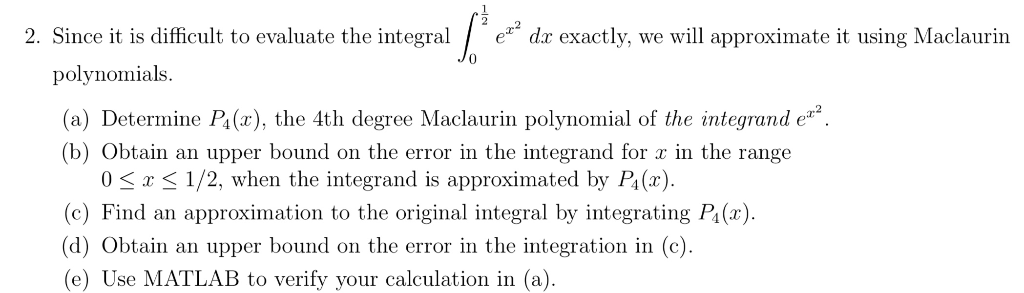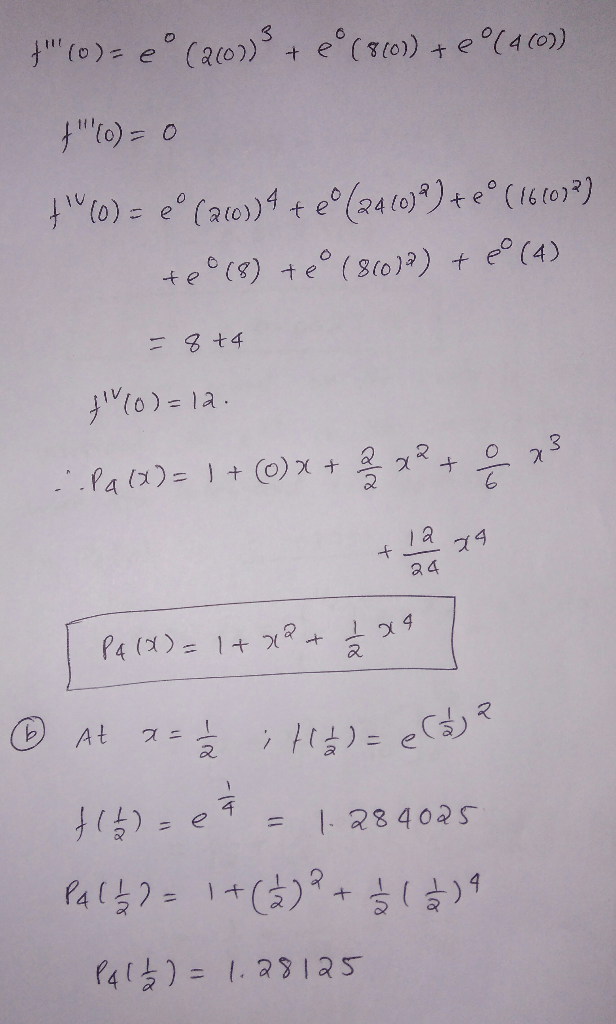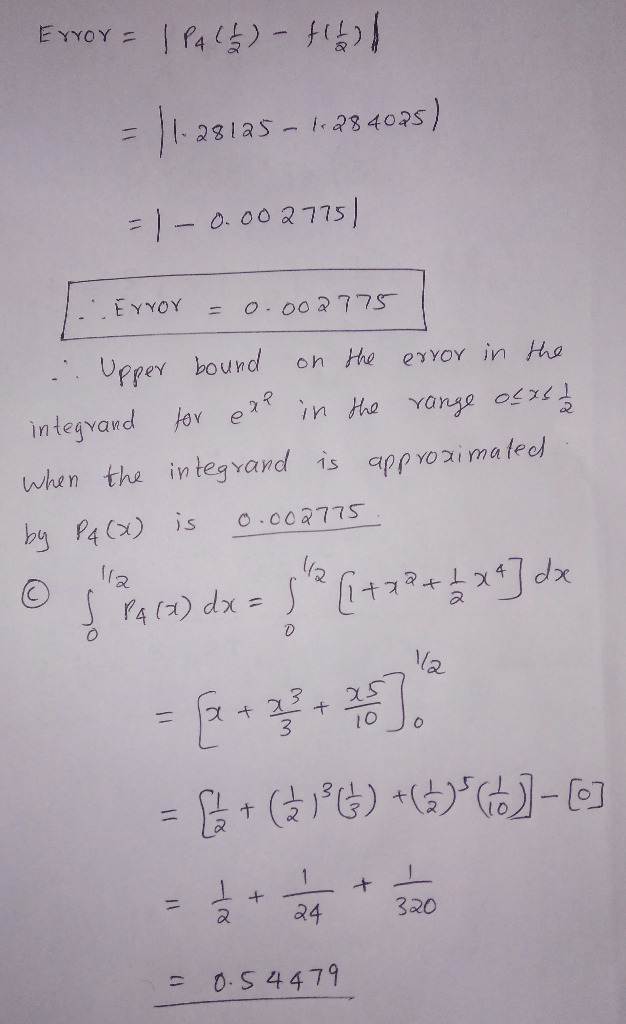# 2. Since it is difficult to evaluate the integral / e dx exactly, we will approximate it using Maclaurin 0 polynomials...2. Since it is difficult to evaluate the integral / e dx exactly, we will approximate it using Maclaurin 0 polynomials (a) Determine Pa(x), the 4th degree Maclaurin polynomial of the integrand e (b) Obtain an upper bound on the error in the integrand for a in the range 0 S x 1/2, when the integrand is approximated by Pi (r) (c) Find an approximation to the original integral by integrating Pa(x) (d) Obtain an upper bound on the error in the integration in (c) (e) Use MATLAB to verify your calculation in (a)##### Add Answer of: 2. Since it is difficult to evaluate the integral / e dx exactly, we will approximate it using Maclaurin 0 polynomials...
More Homework Help Questions Additional questions in this topic.